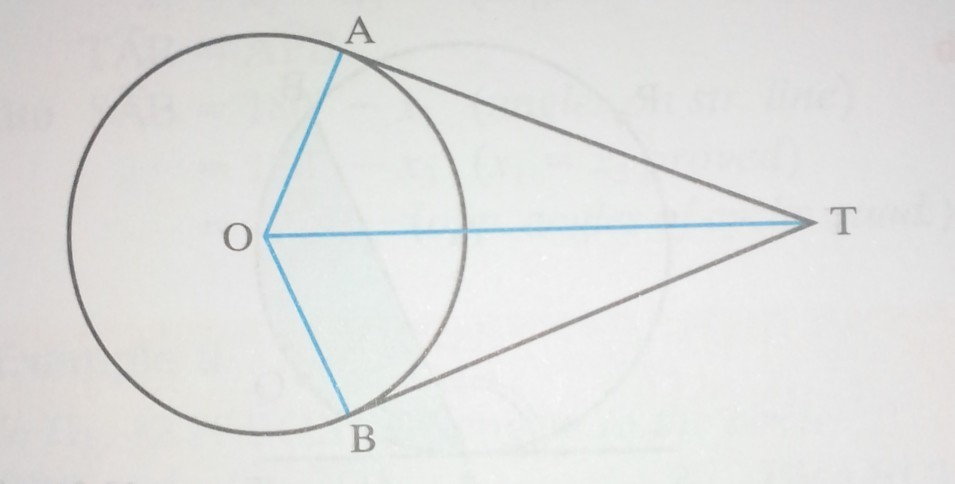### Circle Theorems 2

Mathematics SSS 2 Second Term

WEEK 10

Circle Theorems 2

Performance Objectives

Students should be able to;

1. Prove that the angles in the same segment of a circle are equal
2. Angles in a semicircle is a right angle
3. The opposite angles in a cyclic quadrilateral are supplementary
4. The exterior angle is equal to interior opposite angles

Tangents from an external point

Content

The tangents to a circle from an external point are equal.

Given: a point T outside a circle, centre O, TA and TB are tangents to the circle at A and B.To prove: /TA/ = /TB/.